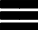Krivalar TutorialsKrivalar Tutorials

# Java Multithreading - join() method

## Java Multithreading - join method

When join() is invoked on a particular thread, the current thread waits for the invoked thread to terminate. Once the invoked thread dies, the current thread continues its execution

Consider the below example when multiple threads execute and join() method is NOT used

``````public class ThreadWithoutJoin implements Runnable{
int sum=0;

}
public void run(){
try{
}
catch(InterruptedException ie){
ie.printStackTrace();
}
for(int i=0;i<1000;i++){
sum=sum+i;
}
}

public void printSum(){

}

public static void main(String a[]) {

te1.printSum();
te2.printSum();
te3.printSum();
te4.printSum();

}

}
``````

```Thread1:0
```

In the above example, main thread terminates before the 4 invoked threads thread1, thread2, thread3 and thread4 complete calculating the sum and hence the result expected is not printed. If Thread join() method is called on each of the instance, the main thread waits until each of invoked instance completes the calculation and terminates.

``````public class ThreadJoinExample implements Runnable{
int sum=0;

}
public void run(){
try{
}
catch(InterruptedException ie){
ie.printStackTrace();
}
for(int i=0;i<1000;i++){
sum=sum+i;
}
}

public void printSum(){

}

public static void main(String a[]) {

try {

} catch (InterruptedException e) {
// TODO Auto-generated catch block
e.printStackTrace();
}

te1.printSum();
te2.printSum();
te3.printSum();
te4.printSum();
}

}
``````

#### Output - MultiThreading using join

```Thread1:499500
```